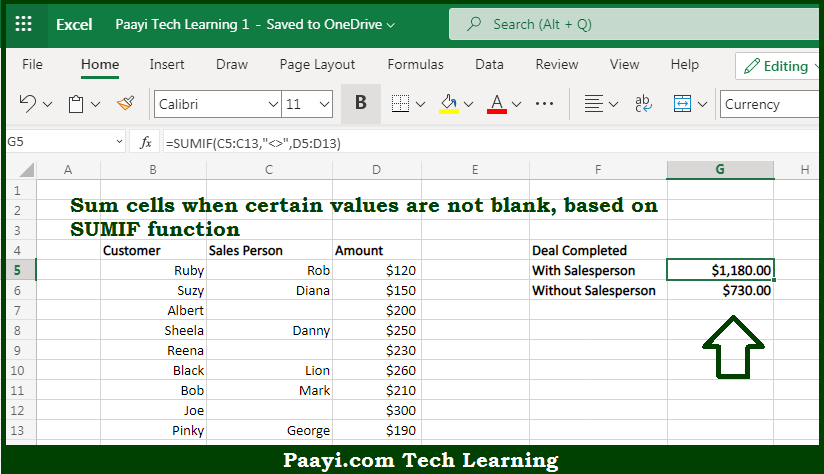# Learn How to SUM If Not Blank in Microsoft Excel

Written by | 0 Comments | 505 Views

In this article, you will learn how to SUM various things in Microsoft Excel using a single/combination(s) of functions. You will also know how to SUM If Not Blank and see the generic formula.

SUM If Not Blank in Microsoft Excel

The main purpose of this formula is to sum cells when certain values are not blank. Here we will learn how to sum if the cell is not blank in the workbook in Microsoft Excel. That implies, with the help of a formula based on the SUMIF function you can able to um cells when certain values are not blank. So, with the help of this formula, you can able to sum if the cell is not blank in the workbook in Microsoft Excel.

General Formula to SUM If Not Blank

=SUMIF(range,"<>",sum_range)

The Explanation for the SUM If Not BlankSo we know that with the help of the given formula above you can able to sum cells when certain values are not blank. Here we will learn how to sum if the cell is not blank in the workbook in Microsoft Excel. As we know that the SUMIF function supports  logical operators efficiently, which are -  "=",">",">=", etc. It should be noted that while using an operator in the criteria for a function like SUMIF, you need to enclose it in double quotes (" "). When you use only "<>" in criteria. That is why there is no need to enter the sum range as a final argument. So, with the help of this formula, you can able to sum if the cell is not blank in the workbook in Microsoft Excel.